# Reflection group

(diff) ← Older revision | Latest revision (diff) | Newer revision → (diff)

A discrete group of transformations generated by reflections in hyperplanes. The most frequently studied are those consisting of mappings of a simply-connected complete Riemannian manifold of constant curvature, i.e. of a Euclidean space, a sphere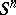or a hyperbolic (Lobachevskii) space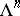.

The theory of reflection groups has its origin in research into regular polyhedra and regular partitions of the Euclidean plane and the sphere ( "ornamentornaments" ). In the second half of the 19th century, this research was extended to include both the-dimensional case, and, in connection with problems of the theory of functions of a complex variable, the hyperbolic plane; regular partitions of the spaceinto regular polyhedra were also described. The symmetry group of any regular polyhedron, as well as the symmetry group of a regular partition of space into regular polyhedra are reflection groups. In 1934 (see ), all reflection groups inandwere enumerated (those incan be considered as a particular case of reflection groups in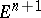). As early as 1925–1927, in the work of E. Cartan and H. Weyl, reflection groups appeared as Weyl groups (cf. Weyl group) of semi-simple Lie groups. It was subsequently established that Weyl groups are in fact those reflection groups in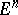that have a single fixed point and are written in a certain basis by integer matrices, while affine Weyl groups are all reflections groups inwith a bounded fundamental polyhedron (see Discrete group of transformations).

## Basic results of the theory of reflection groups.

Let,or. Every reflection group in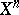is generated by reflectionsin hyperplanes,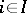, which bound a fundamental polyhedron. Relative to this system of generators, the reflection group is a Coxeter group with defining relations, where the numbersare obtained as follows: If the faces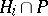and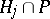are adjacent and the angle between them is equal to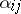, then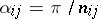; if they are not adjacent, then(and the hyperplanes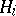and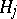do not intersect). On the other hand, any convex polyhedron inall dihedral angles of which are submultiples ofis the fundamental polyhedron of the group generated by the reflections in its bounding hyperplanes.

Every reflection group in(as a group of motions) is the direct product of a trivial group operating in a Euclidean space of a certain dimension, and groups of motions of the following two types:

a finite reflection group whose fundamental polyhedron is a simplicial cone; and (II) an infinite reflection group whose fundamental polyhedron is a simplex. A group of type

can be seen as a reflection group on a sphere with its centre at the vertex of the fundamental cone; its fundamental polyhedron will then be a spherical simplex. A reflection group of type

is uniquely defined by its Coxeter matrix, for which reason the classification of these groups coincides with the classification of finite Coxeter groups. A reflection group of type (II) is defined by its Coxeter matrix up to a dilatation. The classification of these groups, up to a dilatation, coincides with the classification of indecomposable parabolic Coxeter groups. Every reflection group inwith a bounded fundamental polyhedron (as a group of motions) is the direct product of groups of type (II).

Reflection groups inhave been significantly less studied. For many reasons, it is natural to distinguish those whose fundamental polyhedron is bounded or tends to the absolute (the "sphere at infinity" ) only at a finite number of points (this is equivalent to finiteness of the volume). Only these groups are considered below. They are described more or less clearly only for.

A reflection group inis defined by a-gon with angles(if a vertex is infinitely distant, then its angle is considered to be equal to zero). A polygon with such given angles always exists and depends onparameters.

When, the fundamental polyhedron of a reflection group inis uniquely defined by its combinatorial structure and its dihedral angles. For, an exhaustive description of these polyhedra has been obtained

and, thereby, of reflection groups as well. For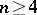, only examples and a few general methods of construction for reflection groups inare known (see , ). It is not known (1990) whether there exists a reflection group inwith a bounded fundamental polyhedron whenand with a fundamental polyhedron of finite volume when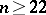.

Linear reflection groups, acting discretely in an open convex cone of a real vector space, are considered alongside reflection groups in spaces of constant curvature. This makes a geometric realization of all Coxeter groups with a finite number of generators possible (see , ).

Every finite reflection group can be seen as a linear group. Of all finite linear groups, finite reflection groups are characterized by the fact that the algebras of invariant polynomials of these groups possess algebraically independent systems of generators . For example, for the group of all permutations of the basis vectors, these will be the elementary symmetric polynomials. Letbe the degrees of the generators of the invariants of a finite reflection group(is the dimension of the space); the numbersare called the exponents of the group. The formula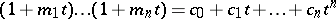holds, where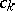is the number of elements infor which the space of fixed points has dimension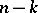. In particular,is equal to the number of reflections in;is equal to the order of the group. Ifis irreducible, then the eigenvalues of its Killing–Coxeter element (see Coxeter group) are equal to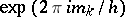, whereis the Coxeter number: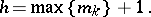The assertions of the previous paragraph, with the exception of the last, also apply to linear groups over an arbitrary field of characteristic zero (see ). In this case it is appropriate to understand a reflection to be a linear transformation with space of fixed points of dimension. All finite linear reflection groups over the field of complex numbers are listed in . Finite linear reflection groups over fields of non-zero characteristic have been found .

How to Cite This Entry:
Reflection group. Encyclopedia of Mathematics. URL: http://encyclopediaofmath.org/index.php?title=Reflection_group&oldid=19056
This article was adapted from an original article by E.B. Vinberg (originator), which appeared in Encyclopedia of Mathematics - ISBN 1402006098. See original article